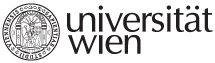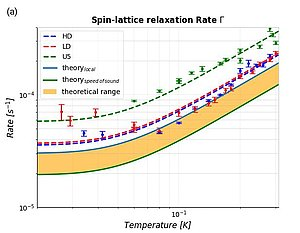﻿ Vienna Computational Materials Laboratory » single_view_scientific_highlightsMonday, 19. February 2018 09:47 Age: 5 yrs

## Solid-state electron spin lifetime limited by phononic vacuum modes

Category: Scientific Highlights

Published in Nature Materials

We investigate on the fundamental mechanism of spin phonon coupling in the negatively charged nitrogen vacancy center (NV) in diamond in order to calculate the spin lattice relaxation time T1 and its temperature dependence ab initio. Starting from the dipolar spin-spin interaction between two electrons, we couple the spins of the electron to the movements of the ions and end up with an effective spin-phonon interaction potential Vs−ph. Taking this time dependent potential as a perturbation of the system leads to Fermi’s golden rule for transition rates which allow to calculate the spin lattice relaxation time T1. We simulate the color center with the Vienna Ab Initio Simulation Package (VASP) to extract the figures necessary to quantify T1: First, we obtain the equilibrium positions of the ions and the electronic charge density by a proper relaxation of the system. Next, we investigate on the local phonon modes of the color center within the harmonic approximation using the small displacement method. We extract the phononic density of states and bandstructure by diagonalizing the dynamical matrix using the phononpy package. Then, we model the electronic wavefunctions by calculating maximally localized Wannier functions with Wannier90 and building the orbitals according to the C3v symmetry of the defect. Finally, we plug the simulated figures into our model and end up with a calculated relaxation time T1 that is very close to the experimental observations.
This Figure shows the measured spin-lattice relaxation times as points for 3 different samples and an interpolated least squares fit for their temperature dependence (dashed lines). The theoretical result depends on the phononic density of states: The green solid line denotes the calculated relaxation rate, if a Debye-model with the calculated velocity of sound in diamond is applied to calculate the density of states, while the light blue line depicts the case, where the k-dependence of the sound velocity was calculated ab-initio and therefore represents the density of states for a crystal consisting of a periodic continuation of the simulated cell.This Figure shows the measured spin-lattice relaxation times as points for 3 different samples and an interpolated least squares fit for their temperature dependence (dashed lines). The theoretical result depends on the phononic density of states: The green solid line denotes the calculated relaxation rate, if a Debye-model with the calculated velocity of sound in diamond is applied to calculate the density of states, while the light blue line depicts the case, where the k-dependence of the sound velocity was calculated ab-initio and therefore represents the density of states for a crystal consisting of a periodic continuation of the simulated cell.

Original publication: T. Astner, J. Gugler, A. Angerer, S. Wald, S. Putz, N. J. Mauser, M. Trupke, H. Sumiya, S. Onoda, J. Isoya, J. Schmiedmayer, P. Mohn, and J. Majer.

Nature/Nature Materials doi.o g/ 10.1038/ s41563-017-0008-y

VIENNA COMPUTATIONAL MATERIALS LABORATORY

Sensengasse 8/12
A-1090 Vienna
AUSTRIA
T: +43-1-4277-51401
F: +43-1-4277-9514
E-Mail
University of Vienna | Universitätsring 1 | 1010 Vienna | T +43-1-4277-0
Lastupdate: 29.09.2014 - 12:13